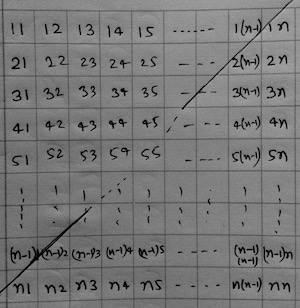##### Calculate the size of a square matrix (n x n) where the elements are present at (i + j) >= n+1.

Calculate the size of a square matrix (n x n) where the elements are present at (i + j) >= n+1.

Pritam Prasun
13 Aug 2017 10:34 pm

This is a simple one.

when you draw an  n x n matrix like below:So, you have (1+ 2 + 3 + 4 + .... n-1 + n = ) n(n+1)/2 places where you have elements present. How the size depends on the data type being stored in the array.

Hence size = n(n+1)/2 * sizeof(data_type)

set2018
13 Aug 2017 10:53 pm

wht does it mean  (i + j) >= n+1

shivani
14 Aug 2017 01:39 am

this is the condition that index of ith row and jth column is greater than or equal to (n+1)

set2018
14 Aug 2017 07:42 am

@shivani

if i =1 and j=1 and if we have 3*3 matrix than

1+1>=3 not possible

1+2>=3 wht does it mean in terms of elements

shivani
14 Aug 2017 11:55 am

you can see it as how many elements in each row satisfy the given condition and sum up all the elements.

Ex. in 1st column 1 element satisfies , in 2nd column 2 elements satisfy ,... likewise for nth column n elements satisfy.
so , its ∑n = n(n+1)/2